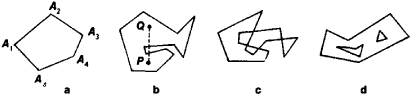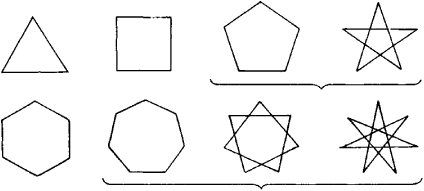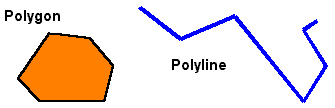# polygon

(redirected from Polysticks)
Also found in: Dictionary, Thesaurus.

## polygon,

closed plane figure bounded by straight line segments as sides. A polygon is convex if any two points inside the polygon can be connected by a line segment that does not intersect any side. If a side is intersected, the polygon is called concave. In a regular polygon the sides are of equal length and meet at equal angles; all other polygons are not regular, although either their sides or their angles may be equal, as in the cases of the rhombus and the rectangle. The simplest regular polygons are the equilateral triangletriangle,
in mathematics, plane figure bounded by three straight lines, the sides, which intersect at three points called the vertices. Any one of the sides may be considered the base of the triangle.
, the squaresquare,
closed plane figure bounded by four straight line segments of equal length and meeting at right angles. The points of intersection of the lines, or sides, are called vertices.
, the regular pentagon (of 5 sides), and the regular hexagon (of 6 sides). Although the Greeks had developed methods of constructing these four polygons using only a straightedge and compass, they were unable to do the same for the regular heptagon (of 7 sides). In the 19th cent. C. F. Gauss showed that a regular heptagon was impossible to construct in this way. He proved that a regular polygon is constructible with a straightedge and compass only when the number of sides p is a prime number (see number theorynumber theory,
branch of mathematics concerned with the properties of the integers (the numbers 0, 1, −1, 2, −2, 3, −3, …). An important area in number theory is the analysis of prime numbers.
) of the form p = 22n + 1 or a product of such primes. The first five regular polygons with a prime number of sides that can be constructed using a straightedge and compass have 3, 5, 17, 257, and 65,537 sides.
The following article is from The Great Soviet Encyclopedia (1979). It might be outdated or ideologically biased.

## Polygon

a closed broken line. More precisely, a polygon is a curve consisting of n line segments that connect arbitrary points A1, A2, A3 …, An−1, An in such a way that every point is connected with the next one and the last point with the first one (see Figure l,a). The points A1, A2 … , An are said to be the vertices of the polygon, and the segments A1, A2, A2, A3 …, AnAn−1An, An A2 are its sides. Furthermore, only plane polygons are considered (that is, it is assumed that a polygon lies in one plane). A polygon can intersect itself (see Figure l,c); moreover, the crunodes, or points of self-intersection, do not necessarily have to be vertices of the polygon.

There exist other definitions of the term “polygon.” A polygon may be said to be a connected part of the plane whose boundary consists of a finite number of line segments, called its sides. In this case, the polygon can also be a multiply connected part of the plane (see Figure l,d), that is, such a polygon can have “polygonal holes.” Infinite polygons, which are parts of the plane bounded by a finite number of line segments and a finite number of half-lines, are also considered.

The ensuing discussion is based on the first definition of “polygon” given above.Figure 1

If a polygon does not cross itself (for example, Figure 1, a and b), then it divides the set of all points of the plane that do not lie on it into two parts—a finite (interior) part and an infinite (exterior) part in the sense that two points belonging to the same part can be connected by a polygonal curve that does not cross the polygon, while if the two points belong to different parts, they cannot be so connected. This fact, known as the Jordan theorem for polygons, seems obvious. Nevertheless, its rigorous deduction from the axioms of geometry is quite difficult. The part of the plane that is interior to a polygon has a definite area. If a polygon crosses itself, it cuts the plane into a number of regions, one of which is infinite (said to be exterior to the polygon) while the rest (known as the interior regions) are finite and simply connected. The boundary of each of the finite regions is a polygon that is free of self-intersections and whose sides are either sides or parts of sides of the given polygon, and whose vertices are vertices or crunodes of that polygon.

If each side of a polygon is assigned a direction, that is, if we indicate which of the vertices is to be considered the beginning of the side and which the end, and, moreover, if the beginning of each side is the end of the preceding side, then we obtain a closed polygonal path, or oriented polygon. The area of the region bounded by an oriented (possibly self-intersecting) polygon is said to be positive if traversal of its boundary in the direction implied by its orientation is counterclockwise (equivalently if the interior of the polygon is invariably on the left side of the oriented boundary) and negative otherwise. Now consider an oriented, possibly self-intersecting, polygon. Draw a line segment from a point in the part of the plane exterior to the polygon to a point in the interior of one of its interior regions. If the polygon crosses this segment p times from the left to the right and q times from the right to the left, then the number p — q (positive or negative integer or zero) is independent of the choice of the exterior point and is said to be the coefficient of this interior region. The sum of the ordinary areas of the interior regions multiplied by their coefficients is taken as the “area” of the given closed path (oriented polygon). The area of a closed path thus defined plays an important role in the theory of mathematical devices (planimeter and others). It is usually expressed in the form of integrals (p2dѡ/2) in polar coordinates p, ∮ or ∮y dx in Cartesian coordinates x, y, where the end of the radius vector p or ordinate y traverses the path once.

The sum of the interior angles of any nonself-intersecting polygon with n sides is equal to (n — 2)180°. A polygon is said to be convex (see Figure l,a) if none of the lines determined by its sides cuts the polygon into two parts. A convex polygon can also be characterized by the property that a line segment connecting any two points of the plane lying within the polygon does not cross the polygon. Every convex polygon is nonself-intersecting but not conversely. For example, Figure l,b depicts a non-self-intersecting polygon that is not convex, since the segment PQ connecting two of its interior points crosses the polygon.

The most important polygons are triangles—in particular, right, isosceles, and equilateral triangles—and quadrangles—in particular, trapezoids, parallelograms, rhombuses, rectangles, and squares. A convex polygon is said to be regular if all its sides are equal and if all its interior angles are equal. Starting with a given side or the radius of the circumscribed circle and using straightedge and compass, the mathematicians of antiquity could construct regular polygons only if the number m of sides was equal to 3 × 2n, 4 × 2n, 5 × 2n , and 3 × 5 × 2n, where n is any positive number or zero. The German mathematician K. Gauss proved in 1801 that it is possible to construct a regular polygon using straightedge and compass if the number of its sides is given by m = 2n × p1 × p2 × … × pk, where p1p2, … are different prime numbers of the form p = 22S + 1 (s is a positive integer). Only five such p are now known: 3, 5, 17, 257, and 65,537. It follows from Galois’ theory that no regular polygons other than those described by Gauss can be constructed by compass and straightedge alone. Thus, construction is possible for m = 3, 4, 5, 6, 8, 10, 12, 15, 16, 17, 20, 24, 32, 34, … and is impossible for m = 7, 9, 11, 13, 14, 18, 19, 21, 22, 23, 25, 26, 27, 28, 29, 30, 31, 33, … .

Table 1 gives the radius of the circumscribed circle, the radius of the inscribed circle, and the area of a regular n-gon (for n = 3, 4, 5, 6, 8, 10) whose side is equal to k.Figure 2

Beginning with the pentagon, there exist nonconvex (self-intersecting, or star-shaped) regular polygons, that is, polygons all of whose sides are equal and each of whose consecutive sides turns in the same direction and by the same angle as its precedent. All the vertices of such polygons also lie on the same circle. One example of such a polygon is the five-pointed star. Figure 2 depicts all the regular, both convex and nonconvex, polygons —from the triangle to the heptagon.

## polygon

[′päl·i‚gän]
(mathematics)
A figure in the plane given by points p1, p2, …, pn and line segments p1 p2, p2 p3, …, pn-1 pn , pn p1.
McGraw-Hill Dictionary of Scientific & Technical Terms, 6E, Copyright © 2003 by The McGraw-Hill Companies, Inc.

## polygon

a closed plane figure bounded by three or more straight sides that meet in pairs in the same number of vertices, and do not intersect other than at these vertices. The sum of the interior angles is (n--2) × 180° for n sides; the sum of the exterior angles is 360°. A regular polygon has all its sides and angles equal. Specific polygons are named according to the number of sides, such as triangle, pentagon, etc.
Collins Discovery Encyclopedia, 1st edition © HarperCollins Publishers 2005

## polygon

In computer graphics, a multi-sided object that can be filled with color or moved around as a single entity. See triangle.Copyright © 1981-2019 by The Computer Language Company Inc. All Rights reserved. THIS DEFINITION IS FOR PERSONAL USE ONLY. All other reproduction is strictly prohibited without permission from the publisher.
Site: Follow: Share:
Open / Close